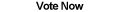Home Articles Components Code Snippets Links# C# Optimal Five-Element Sort

## C# Sorting

Sorting in C# (and in virtually any programming language) is an essential part of application programming. Sorting in general is one of the most well-studied topics in computer science. In this article we'll explore a very small, but interesting application of sorting algorithms.

While the sorting algorithm presented will seem trivial, it does have useful applications.

## Comparsion-Based Sorting

This particular sorting algorithm is comparison-based. A comparsion-based sorting algorithm is simply an algorithm that puts elements in order by comparing them to each other. This type of sorting is very important because it works for absolutely any type of elements that can be compared.

What's a non-comparison-based sorting algorithm? Bucket sort is an example. Bucket sort is a powerful and fast sorting algorithm. However it only works on numeric data. For C# that means integers, floats, decimals, etc.

So you can see the advantage of a more generalized sorting algorithm. A comparison-based algorithm is flexible in the types of objects it can sort. There are many types of sorting algorithms that work this way. The faster ones are Mergesort, Quicksort, and Heap Sort. Slower ones are Insertion Sort and Selection Sort. (Bubble sort is even slower).

How can we tell these are fast? We can compare the performance of different sorting algorithms by running actual tests on data. We can have a function sort a set of 20 elements 10,000 times and see how long it takes. But this leaves a lot of factors open, such as the programming language used, the computer used to run the program, etc. A much cleaner way is to use the number of comparisons an algorithm needs in the worst case to sort a set of objects.

## Optimal Sorting

A bit of theoretical analysis tells us that comparsion-based algorithms can never run faster than O(n log n) asymptotically. Or in more precise terms means that any comparsion-based algorithm cannot do less than the ceiling of lg(n!) number of comparisons.

In other words, algorithms might take more comparisons to come up with the right answer (a sorted list), but no matter how clever, there is a minimum number of comparisons needed to guarantee the right answer.

For a 5 element sort, we can plug in 5 into our little formula:

`Ceiling(lg(5!)) = Ceiling(lg(120)) = Ceiling(6.9...) = 7`

The least, or optimal, number of comparisons to correctly sort 5 objects is 7 comparisons.

So why exactly are we looking at 5 elements? Five elements is where even the asymptotically optimal sorting algorithms (such as Mergesort) start to do more comparisons than the optimal amount required.

So in this case, our five-element sorting algorithm will be the fastest sorting algorithm possible, in terms of number of comparisons.

### Page 2Five-Element Sorting Algorithm >>

Back to C# Article List

Other C# Articles